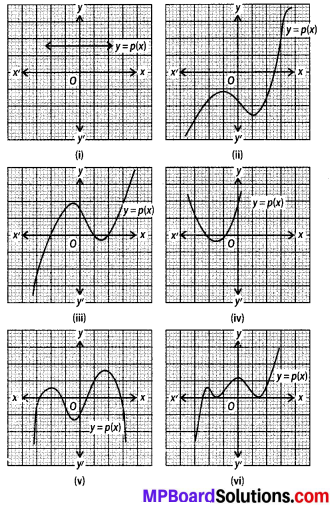In this article, we will share MP Board Class 10th Maths Book Solutions Chapter 2 Polynomials Ex 2.1 Pdf, These solutions are solved subject experts from the latest edition books.

## MP Board Class 10th Maths Solutions Chapter 2 Polynomials Ex 2.1Question 1.
The graphs of y = p(x) are given in the below figures, for some polynomials p(x). Find the number of zeroes of p{x), in each case.Solution:
(i) The given graph is parallel to x-axis, it does not intersect the x-axis.
It has no zero.
(ii) The given graph intersects the x-axis at one point only.
∴ It has one zero.
(iii) The given graph intersects the x-axis at three points.
∴ It has three zeroes.
(iv) The given graph intersects the x-axis at two points.
∴ It has two zeroes.
(v) The given graph intersects the x-axis at four points.
∴ It has four zeroes.
(vi) The given graph meets the x-axis at three points.
∴ It has three zeroes.